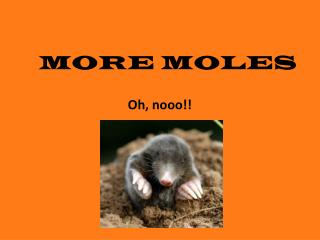Download PresentationMORE MOLES

# MORE MOLES - PowerPoint PPT PresentationDownload Presentation## MORE MOLES

- - - - - - - - - - - - - - - - - - - - - - - - - - - E N D - - - - - - - - - - - - - - - - - - - - - - - - - - -
##### Presentation Transcript

1. MORE MOLES Oh, nooo!!

2. A SMALL REVIEW • How many protons, neutrons, and electrons are in each of the following: • 2412Mg i) 3517Cl • 2412Mg+2 j) 3517Cl- • 5927Co+2 k) 3216S-2 • 5927Co+3 l) 3216S • 5927Co • 7934Se • 7934Se+2 • 6328Ni+2

3. Would you expect each of the following atoms to lose or gain electrons? • Ra • In • P • S • Br • Rb

4. Name the compounds: • NaBr • Rb2O • CaS • AlI3

5. Write the formulas for the following compounds: • Strontium fluoride • Aluminum selenide • Potassium nitride • Magnesium phosphide • Tin (II) nitride • Cobalt (II) chloride • Mercury (II) oxide • Chromium (VI) sulfide

6. WHICH ELEMENT IN THE PERIODIC TABLE WOULD HAVE THE LOWEST IONIZATION POTENTIAL? WHY? WHICH ELEMENT IN THE PERIODIC TABLE WOULD HAVE THE HIGHEST IONIZATION POTENTIAL? WHY? WHICH ELEMENT WOULD HAVE THE HIGHEST ELECTRON AFFINITY? WHY? WHICH ELEMENT WOULD HAVE THE LOWEST ELECTRON AFFINITY? WHY?

7. NAME THE FOLLOWING COMPOUNDS: • N2O5 • SO3 • P4S5 • SeF6 • B2Si • NF3

8. WHAT IS AN IONIC BOND? WHAT IS A COVALENT BOND? WHAT IS A POLAR COVALENT BOND? WHAT IS A METALLIC BOND?

9. WHAT DOES ELECTRONEGATIVITY MEASURE? HOW COULD YOU USE IT TO DECIDE IF A BOND BETWEEN THE ATOMS OF TWO ELEMENTS WOULD BE COVALENT, IONIC, OR POLAR COVALENT?

10. ELECTRONEGATIVITY IS A MEASURE OF THE ATTRACTION FOR A SHARED PAIR OF ELECTRONS BETWEEN TWO ATOMS IN A CHEMICAL BOND. VALUES CAN RANGE FROM 0.7 TO 3.98. LITTLE OR NO ELECTRONEGATIVITY DIFFERENCE RESULTS IN A PURE COVALENT BOND. A SMALL DIFFERENCE RESULTS IN A POLAR COVALENT BOND. A LARGE DIFFERENCE RESULTS IN AN IONIC BOND.

11. ATOMS WITH THE SAME OR SIMILAR ELECTRONEGATIVITIES WOULD FORM PURE COVALENT BONDS. • THE RULES ARE: • Non-polar bonds occur when the difference in electronegativity between the two atoms is less than 0.4 • Polar bonds occur when the difference in electronegativity between the two atoms is between 0.4 and 1.7 • Ionic bonds occur when the difference in electronegativity between the two atoms is greater than 1.7

12. What is a mole? What do we mean by molar mass? Formula mass? What is the difference between the empirical formula and the molecular formula of a substance? How many years are in 1 mole of seconds?

13. HOW MANY MOLES ARE IN 48 g OF AlPO4? HOW MANY GRAMS ARE IN 3.2 MOLES OF KNO3 ? HOW MANY MOLECULES ARE IN 4 MOLES OF H2O? HOW MANY grams ARE IN 8.7 X 1026 MOLECULES OF N2O5?

14. WHAT KIND OF BONDS DOES CARBON FORM? WHY? WHAT IS MEANT BY CARBON DATING? WHAT IS THE LOW TEMPERATURE AND PRESSURE FORM OF CARBON? WHAT IS THE HIGH TEMPERATURE AND PRESSURE FORM OF CARBON?

15. CAN YOU BURN A DIAMOND? WHY? WHY IS DIAMOND AN INSULATOR AND GRAPHITE AN EXCELLENT CONDUCTOR OF ELECTRICITY? WHY IS CARBON SUCH A UNIQUE ELEMENT?

16. CALCULATE THE NUMBER OF GRAMS OF LiOH FORMED WHEN 20 g LiN3 REACTS WITH WATER. Li3N + H2O  LiOH + NH3

17. HOW MANY MOLES OF DIPHOSPHORUS PENTOXIDE ARE FORMED WHEN 3.25 MOLES OF PHOSPHORUS REACT WITH OXYGEN?